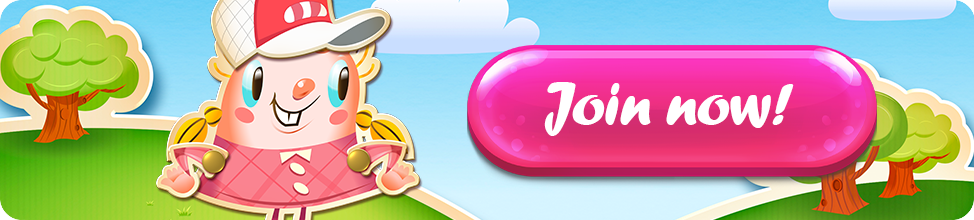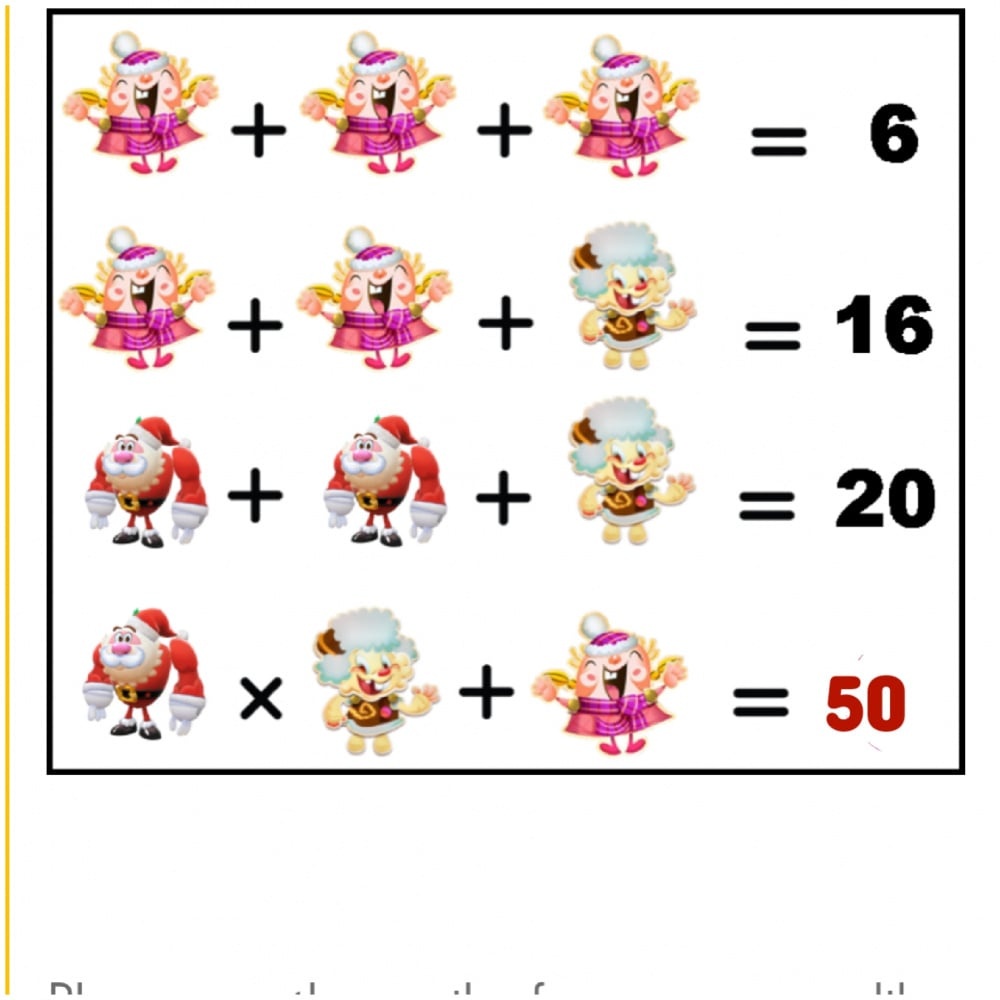# (FINISHED) Win Gold Bars! Christmas Candy Crush Saga Math Contest.

## Comments

• 38

·
• Good luck Candy Crushers

My final answer-50

·
• 4×12=48+2=50 Final answer-50

·
• edited December 2019

50·
• Hi @Elsa and friends..here is my answer in Candy Saga. Thanks.

2 + 2 + 2 = 6
2 + 2 + 12 = 16
4 + 4 + 12 = 20
4 x 12 + 2 = 50

New norms. Avoid 3C. (Crowded place, Confined space & Close conversation) Do 3W. (Wash hands, Wear mask & Warn new norms)

·
• My answer is 50

·
• 2+2+2 = 6
2+2+12 = 16
4+4+12 = 20
4x12+2 = 50
? = 50

·
• 53

·
• The answer is 50.

·
• Aced it
·
• 50!

·
• 38

38
Geben Sie Ihren Kommentar ein
·
• 38
·
•  Spoiler.

50
·
• Answer is 50

·
Sign In or Register to comment.